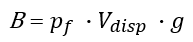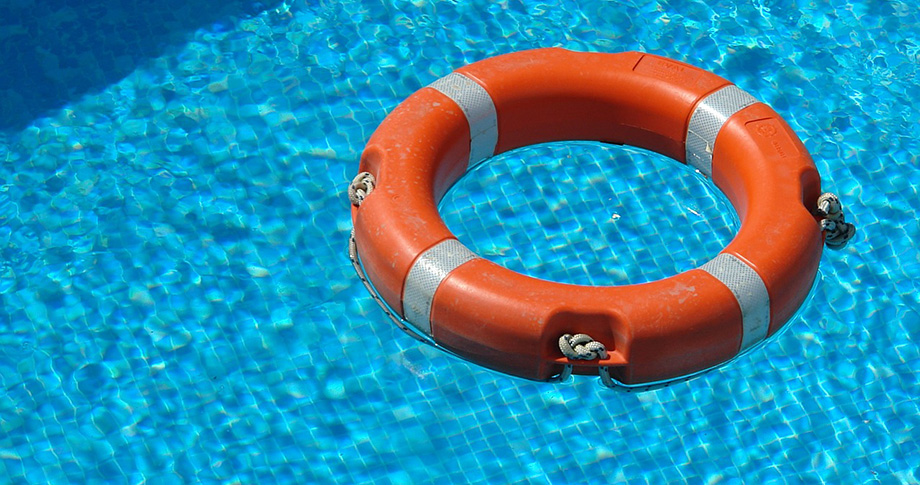# Buoyancy Calculator

Easily calculate the buoyant force experienced by a body / object submerged in a fluid given the fluid's density and the displaced volume. The buoyancy calculator also outputs the weight of the displaced fluid and supports both metric and imperial measurement units. The calculator can be used for both fully-submerged and partially-submerged objects.

Share calculator:

Embed this tool:
get code

## What is a buoyant force?

Buoyancy is an upward force exerted by a fluid in direction opposite to a downward force such as gravity and thus it counters (partially or fully) the weight of an object immersed in the fluid. Pressure increases with depth due to the weight of the increasing quantity of fluid above it so the further down an object has to push its way, the more resistance it faces. This is why if you push an object down slightly and remove your hand, it will float back up due to the greater buoyancy force encountered at the new depth. Similarly, the pressure at the bottom of a submerged object is greater than that at the top of the object which results in a pressure difference responsible for the net upward force on the object we call buoyancy force. The buoyancy principle was famously discovered by Archimedes and can be stated approximately as such: "Any object, wholly or partially immersed in a fluid, is buoyed up by a force equal to the weight of the fluid displaced by the object".

The magnitude of the buoyancy force is measured in Newtons and is equivalent to the weight of the fluid displaced by the object, which is also proportional to the pressure difference. As a result, if a submerged object has greater average density than the fluid it tends to sink ("fall into water"), otherwise the buoyancy force keeps it afloat. Buoyancy exists only as long as there is a force pressing the object into the fluid, e.g. gravity forcing a human body into sea water. Using our buoyant force calculator you can compute both the buoyant force and the displaced fluid mass in tons and tonnes. When the fluid is water it functions as a water displacement calculator (sea-water density is ~1024 kg/m3, fresh water is ~1000 kg/m3, see the fluid density table).

## Buoyant Force Formula

The formula for the buoyant force (a.k.a. buoyancy equation) (B) is expressed in terms of the body's volume or more precisely the volume of fluid displaced by the body Vdisp in m3, the density of the fluid in question (p, in kg/m3) and the gravitational (or equivalent) force g in m/s2 by the equation:The result is the buoyant force in Newtons. Straightforward transformations can be performed to solve for any of the variables. In our calculator you can enter gravity both in m/s2 and as g-units where 1g = 9.80665 m/s2 is the standard acceleration due to Earth's gravity at sea-level.

The formula is valid for homogenous materials where the density of all points in the object equals its total mass divided by its total volume. Determining the density of a gas or liquid may require the use of specialized equipment like a hydrometer, a dasymeter or a Coriolis flow meter. If the object is of heterogeneous density a piece-wise approach may be preferred.

## Calculating the buoyant force - examples

Example 1: A ship has a displacement tonnage (the total weight of the volume of water a ship "displaces" when it is partially-submerged in the water) of 10 tonnes. Let us say it is sailing at sea and we know that sea-water density is approximately 1024 kg/m3, on average. What is the buoyant force experienced by the ship?

First, convert the tonnes to kg by multiplying by 1,000 to get 10,000 kg. Then use the buoyant force equation above to get B = 10,000 · 1024 · 9.80665 = 100,518,162.5 Newtons (N) or around 100.52 MegaNewtons (MN).

Example 2: If the density of water in a swimming pool is 1,000 kg/m3 and we have a life saver which weighs 1kg and has a volume of 0.12 m3, what is the maximum weight of a person so they can safely float using this life preserver? Will a 130 kg person float with it?

In this case we do not even need to know the buoyant force to estimate the maximum weight the life preserver can support on top of its own weight. We simply multiply the volume displaced by the life preserver which is 0.12 m3 (assuming no caving in) by the density of the water to get the total weight which would just barely float: 0.12 · 1,000 = 120 kg. Subtracting the weight of 1 kg and allowing, say, 5-10 kg for comfortable floating such a life preserver should be able to keep afloat in fresh water a person weighing up to 110 kg. To answer the second question - a 130 kg human will not be able to float with it.Our buoyancy calculator will automatically convert for you any units to the base SI units (e.g. kilograms to pounds). All of the above buoyancy calculation examples are just educational ones, obviously.

## Density of water and other common liquids

The density of a material or substance varies with its temperature and pressure, which is typically negligible for solids and liquids but is much greater for gases. Increasing the pressure on a substance decreases its volume and increases its density. Increasing the temperature generally decreases the material density and increases its volume (increasing denominator in the formula). A substance with uneven temperature will also have varying pressure and density.

As an example of how water density changes with temperature, at 1 atm pressure water has density of 983.854 kg/m3 at -30 °C, 999.9720 kg/m3 at 4 °C and only 958.4 kg/m3 at 100 °C (boiling temperature at sea-level). When the substance is a mix, its density changes. Sea-water density is, on average, about 1,025 kg/m3. Here is a reference table for some commonly encountered fluids:

Density of common fluids
FluidDensity (kg/m3)
Air 1
Water 998
Sea-water 1020-1050
Ethanol 789
Alcohol 800
Milk 1020-1050
Plant Oil (Cooking Oil) 910-920
Fuel Oil 890
Auto Oils 880-940

Where ranges are given the density and thus buoyancy varies depending on the particular type of substance or mixture. E.g. average sea-water density is 1024 but it can reach 1050 under certain conditions like high pressure and high concentration of salts.